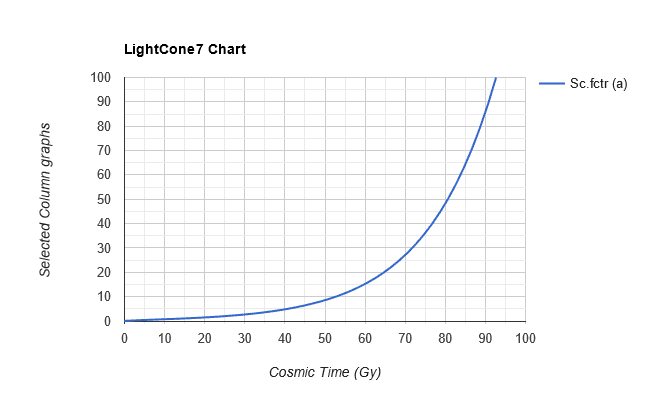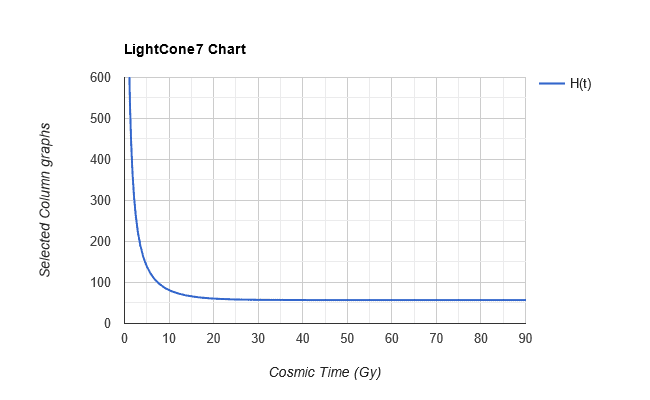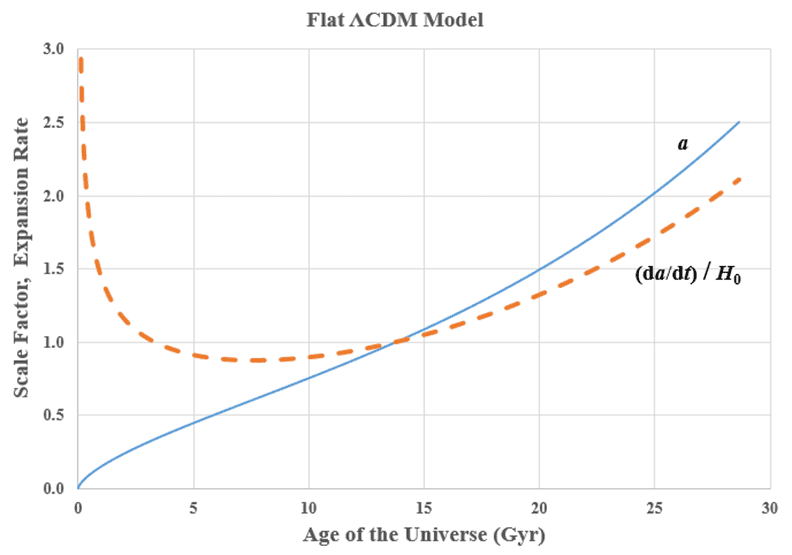# Accelerating expansion of the Universe

• B
• Jupiter60
This is because the scale factor (a) increases without limit, and the Hubble parameter (H) stays constant.f

#### Jupiter60

TL;DR Summary
Expansion
Will the universe keep expanding faster and faster for all eternity or is there a limit to how fast the expansion will get?

In the current model, the expansion is driven by the dark (vacuum) energy. As the universe expands, the vacuum energy becomes ever more dominant and the expansion rate increases without limit.

Last edited:
In the current model, the expansion is driven by the dark (vacuum) energy. As the universe expands, the vacuum energy becomes ever more dominant and the expansion rate increases without limit.
The expansion rate approaches the one corresponding to the vacuum energy. It is decreasing towards that value as total energy density decreases.

The expansion rate approaches the one corresponding to the vacuum energy. It is decreasing towards that value as total energy density decreases.

To clarify the above exchange for the OP: there's a difference between expansion and expansion rate.

In the current model the former continues growing without limit, with distances growing ever faster, and approaching exponential expansion (meaning: any two sufficiently distant galaxies will recede faster and faster).
The latter decreases towards a constant value (meaning: every distance will eventually grow by the same percentage after the same amount of time).
Constant expansion rate equates exponential expansion.

In technical parlance expansion is the growth of the scale factor ##a##, i.e. ##\dot a>0##. While expansion rate is the value of the Hubble parameter ##H##.

Expansion:Expansion rate vs time:••mattt, kimbyd, jim mcnamara and 5 others
What @Bandersnatch said.

•phinds
Summary:: Expansion

Will the universe keep expanding faster and faster for all eternity or is there a limit to how fast the expansion will get?

For models that assume a non-expanding universe, the scale factor, a, remains constant and the expansion rate, da/dt = 0.

In the Big Bang model, a(t) continues to increase and da/dt > 0.

Using Jorrie’s calculator, we can calculate a(t) and the Hubble parameter, H(t) (see Post #5). The expansion rate can be calculated with the equation,

da/dt = a(t) H(t) (see, for example, Hubble's law)​

The results are plotted below:The above figure suggests that, according to the ΛCDM model, the universe will keep expanding faster and faster forever (see Post #2).